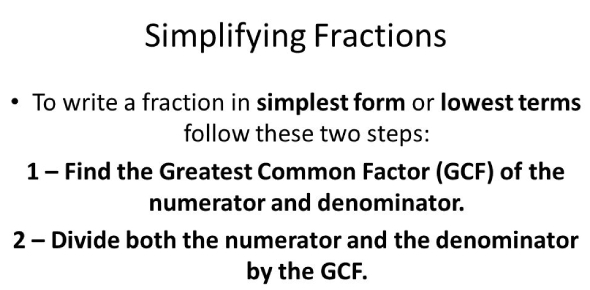# Simplifying Fractions: Math Quiz! Trivia

By Daycel Alon
Daycel Alon, Mathematics
With 20 years of experience, Daycel is a dedicated elementary school teacher, currently focusing on teaching Mathematics to 3rd-grade students. Their enduring commitment to education reflects in the positive impact they have made on young minds throughout their two-decade teaching journey.
Quizzes Created: 1 | Total Attempts: 1,286
, Mathematics
Approved & Edited by ProProfs Editorial Team
The editorial team at ProProfs Quizzes consists of a select group of subject experts, trivia writers, and quiz masters who have authored over 10,000 quizzes taken by more than 100 million users. This team includes our in-house seasoned quiz moderators and subject matter experts. Our editorial experts, spread across the world, are rigorously trained using our comprehensive guidelines to ensure that you receive the highest quality quizzes.
Questions: 10 | Attempts: 1,336Settings.

• 1.

### Simplified 15/40.

• A.

3/8

• B.

1/2

• C.

3/4

• D.

5/8

A. 3/8
Explanation
To simplify the fraction 15/40, we can divide both the numerator and denominator by their greatest common divisor, which is 5. Dividing 15 by 5 gives us 3, and dividing 40 by 5 gives us 8. Therefore, the simplified form of 15/40 is 3/8.

Rate this question:

• 2.

### Which is the lowest term of 6/24?

• A.

1/4

• B.

3/12

• C.

4/6

• D.

3/8

A. 1/4
Explanation
The lowest term of a fraction is when the numerator and denominator have no common factors other than 1. To find the lowest term of 6/24, we need to simplify the fraction. Both 6 and 24 can be divided by 6, so we divide both the numerator and denominator by 6 to get 1/4. Therefore, 1/4 is the lowest term of 6/24.

Rate this question:

• 3.

### Which is the lowest term for 8/48?

• A.

1/6

• B.

4/12

• C.

8/48

• D.

1/2

A. 1/6
Explanation
The lowest term for 8/48 is 1/6 because both the numerator and denominator can be divided by 8, resulting in 1/6.

Rate this question:

• 4.

### 2/5 and 10/25 are equivalent to fractions.

• A.

True

• B.

False

A. True
Explanation
The fractions 2/5 and 10/25 are equivalent because they represent the same value. To check this, we can simplify both fractions. By dividing the numerator and denominator of 10/25 by 5, we get 2/5. Therefore, the fractions 2/5 and 10/25 are equivalent.

Rate this question:

• 5.

### 3/9 cannot be simplified.

• A.

True

• B.

False

B. False
Explanation
The given statement is false because 3/9 can be simplified. Both the numerator and denominator have a common factor of 3, so we can divide both by 3 to simplify the fraction. After simplifying, we get 1/3, which is the simplest form of 3/9.

Rate this question:

• 6.

### 3/4 is the lowest term of 6/12.

• A.

True

• B.

False

B. False
Explanation
The statement is false because 3/4 is not the lowest term of 6/12. To find the lowest term of a fraction, we need to simplify it by dividing both the numerator and denominator by their greatest common divisor. In this case, the greatest common divisor of 6 and 12 is 6. Dividing both the numerator and denominator of 6/12 by 6 gives us 1/2, which is the lowest term of the fraction.

Rate this question:

• 7.

### Which of the following will help reduce fractions into lowest terms?

• A.

Divide the numerator and denominator by their GCF

• B.

Divide the numerator and denominator by their LCM

• C.

Multiply the numerator by its denominator

• D.

Divide the numerator by its denominator

A. Divide the numerator and denominator by their GCF
Explanation
Dividing the numerator and denominator by their greatest common factor (GCF) will help reduce fractions into lowest terms. The GCF is the largest number that divides both the numerator and denominator without leaving a remainder. By dividing both the numerator and denominator by their GCF, we can simplify the fraction and express it in its lowest terms. This process ensures that the fraction cannot be further simplified and is in its simplest form.

Rate this question:

• 8.

### Which of the following is in the simplest form?

• A.

5/6

• B.

3/6

• C.

2/6

• D.

4/6

A. 5/6
Explanation
The fraction 5/6 is in the simplest form because the numerator (5) and the denominator (6) have no common factors other than 1. This means that the fraction cannot be further reduced or simplified.

Rate this question:

• 9.

### Which of the following is the lowest term for 5/55?

• A.

1/11

• B.

5/11

• C.

11/55

• D.

1/55

A. 1/11
Explanation
The lowest term for a fraction is when the numerator and denominator have no common factors other than 1. In this case, the numerator 5 and the denominator 55 have a common factor of 5. By dividing both the numerator and denominator by 5, we get the fraction 1/11, which is the lowest term for 5/55.

Rate this question:

• 10.

### Which of the following is equivalent to 3/12?

• A.

1/4

• B.

3/4

• C.

1/6

• D.

2/5

A. 1/4
Explanation
The fraction 3/12 can be simplified by dividing both the numerator and denominator by their greatest common divisor, which is 3. When we divide 3 by 3, we get 1, and when we divide 12 by 3, we get 4. Therefore, the equivalent fraction of 3/12 is 1/4.

Rate this question:

Daycel Alon |Mathematics
With 20 years of experience, Daycel is a dedicated elementary school teacher, currently focusing on teaching Mathematics to 3rd-grade students. Their enduring commitment to education reflects in the positive impact they have made on young minds throughout their two-decade teaching journey.

Related TopicsBack to top